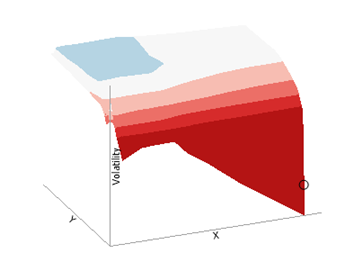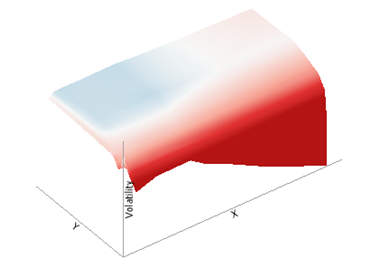Altair® Panopticon

# 3D Surface Plot

3D Surface Plots are a 3D perspective version of the 2D Surface Plot.

They provide a clearer understanding of the overall “shape” of the surface but they also introduce occlusion problems; not all data points can be seen due to the display perspective.

The Surface Plot 3D is made up of a series of points where each point has:

• X Position

• Y Position

• Z Position (encoded by color)

The Surface Plot 3D can support data sets where the X and Y positions can both be regular and irregular in their distribution.

The color scale can be continuous or stepped to show a surface gradient.

Grid lines, a ground plane, and markers for data points can be shown if required.Figure 1. A 3D surface plot with stepped colorsFigure 2. A 3D surface plot with continuous colors